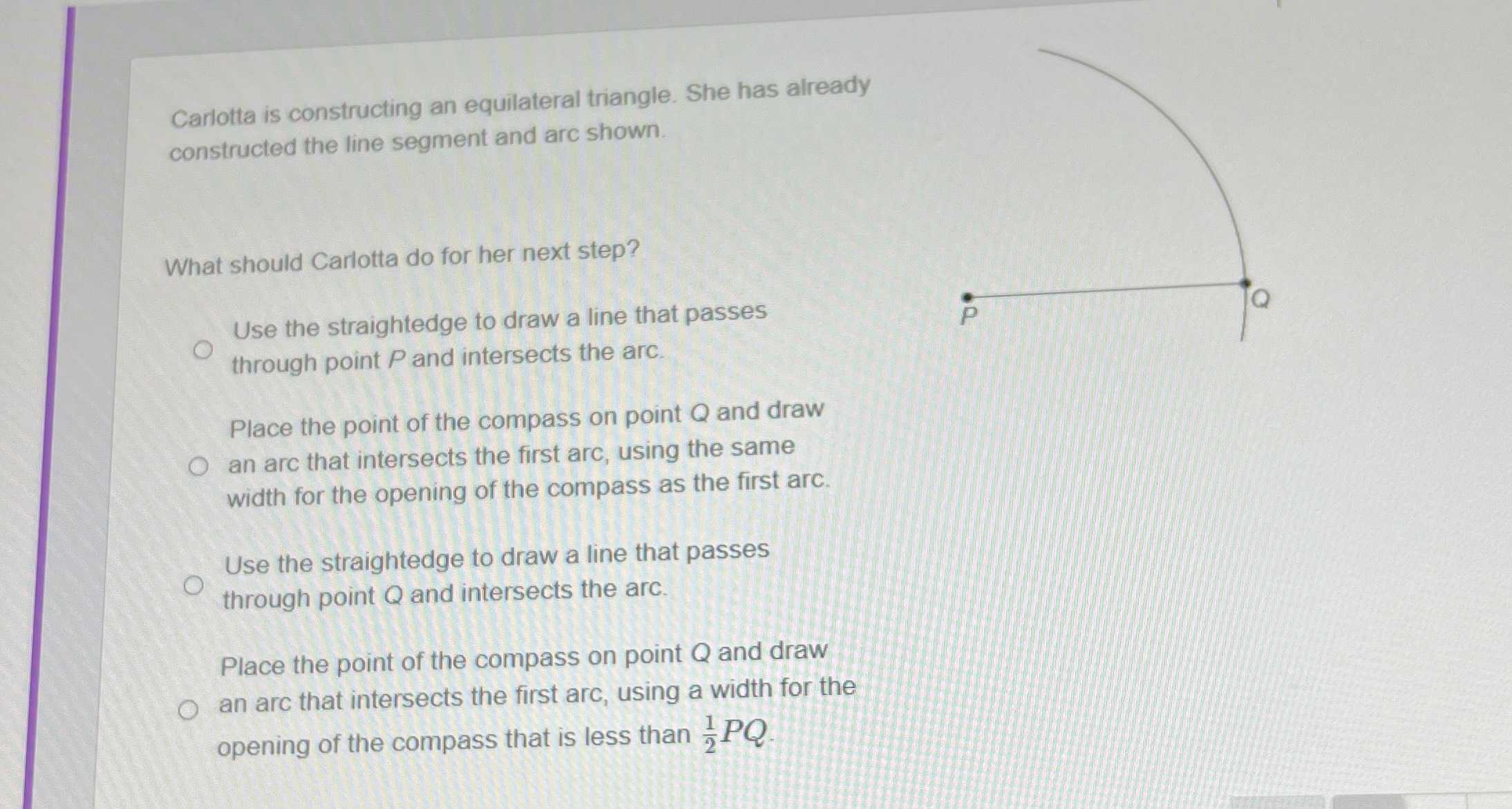### Still have math questions?

Trigonometry
QuestionWhat should Carlotta do for her next step? is constructing an equilateral triangle. She has already constructed the line segment and arc shown.

Use the straightedge to draw a line that passes through point $$P$$ and intersects the arc.

Place the point of the compass on point $$Q$$ and draw intersects the first arc, using the same width for the opening of the compass as the first arc.

Use the straightedge to draw a line that passes through point $$Q$$ and intersects the arc.

Place the point of the compass on point $$Q$$ and draw opening of the compass that is less than $$\frac { 1 } { 2 } P Q .$$